# Black hole thermodynamics

In physics, black hole thermodynamics is the area of study that seeks to reconcile the laws of thermodynamics with the existence of black hole event horizons. Much as the study of the statistical mechanics of black body radiation led to the advent of the theory of quantum mechanics, the effort to understand the statistical mechanics of black holes has had a deep impact upon the understanding of quantum gravity, leading to the formulation of the holographic principle.An artist depiction of two black holes merging, a process in which the laws of thermodynamicsare upheld.

## Black hole

The only way to satisfy the second law of thermodynamics is to admit that black holes have entropy. If black holes carried no entropy, it would be possible to violate the second law by throwing mass into the black hole. The increase of the entropy of the black hole more than compensates for the decrease of the entropy carried by the object that was swallowed.

Starting from theorems proved by Stephen Hawking, Jacob Bekenstein conjectured that the black hole entropy was proportional to the area of its event horizon divided by the Planck area. Bekenstein suggested$(1/2cdotln{2})/4pi$ as the constant of proportionality, asserting that if the constant was not exactly this, it must be very close to it. The next year, Hawking showed that black holes emit thermal Hawking radiation corresponding to a certain temperature (Hawking temperature). Using the thermodynamicrelationship between energy, temperature and entropy, Hawking was able to confirm Bekenstein’s conjecture and fix the constant of proportionality at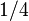$1/4$:$S_{text{BH}} = frac{kA}{4ell_{mathrm{P}}^2}$

where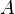$A$ is the area of the event horizon, calculated at$4pi R^2$,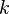$k$ is Boltzmann’s constant, and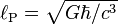$ell_{mathrm{P}}=sqrt{Ghbar / c^3}$ is the Planck length. This is often referred to as theBekenstein–Hawking formula. The subscript BH either stands for “black hole” or “Bekenstein-Hawking”. The black hole entropy is proportional to the area of its event horizon$A$. The fact that the black hole entropy is also the maximal entropy that can be obtained by the Bekenstein bound (wherein the Bekenstein bound becomes an equality) was the main observation that led to the holographic principle.

Although Hawking’s calculations gave further thermodynamic evidence for black hole entropy, until 1995 no one was able to make a controlled calculation of black hole entropy based on statistical mechanics, which associates entropy with a large number of microstates. In fact, so called “no hair” theorems appeared to suggest that black holes could have only a single microstate. The situation changed in 1995 when Andrew Strominger and Cumrun Vafa calculated  the right Bekenstein-Hawking entropy of asupersymmetric black hole in string theory, using methods based on D-branes and string duality. Their calculation was followed by many similar computations of entropy of large classes of other extremal and near-extremal black holes, and the result always agreed with the Bekenstein-Hawking formula. However, for the Schwarzschild black hole, viewed as the most far-from-extremal black hole, the relationship between micro and macro-states is expected to be clarified from the string theoretical viewpoint. Various studies are in progress, but this has not yet been elucidated.

In Loop quantum gravity (LQG) it is possible to associate a geometrical interpretation to the microstates: these are the quantum geometries of the horizon. LQG offers a geometric explanation of the finiteness of the entropy and of the proportionality of the area of the horizon. It is possible to derive, from the covariant formulation of full quantum theory (Spinfoam) the correct relation between energy and area (1st law), the Unruh temperature and the distribution that yields Hawking entropy. The calculation makes use of the notion of dynamical horizon and is done for non-extremal black holes. There seems to be also discussed the calculation of Beckenstein-Hawking entropy from the point of view of LQG.

## The laws of black hole mechanics

The four laws of black hole mechanics are physical properties that black holes are believed to satisfy. The laws, analogous to the laws of thermodynamics, were discovered by Brandon Carter, Stephen Hawking and James Bardeen.

### Statement of the laws

The laws of black hole mechanics are expressed in geometrized units.

#### The Zeroth Law

The horizon has constant surface gravity for a stationary black hole.

#### The First Law

For perturbations of stationary black holes, the change of energy is related to change of area, angular momentum, and electric charge by:$dE = frac{kappa}{8pi},dA+Omega, dJ+Phi, dQ,$

where$E$ is the energy,$displaystyle kappa$ is the surface gravity,$A$ is the horizon area,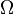$Omega$ is the angular velocity,$J$ is the angular momentum,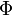$Phi$ is the electrostatic potential and$Q$ is theelectric charge.

#### The Second Law

The horizon area is, assuming the weak energy condition, a non-decreasing function of time,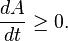$frac{dA}{dt} geq 0.$

This “law” was superseded by Hawking’s discovery that black holes radiate, which causes both the black hole’s mass and the area of its horizon to decrease over time.

#### The Third Law

It is not possible to form a black hole with vanishing surface gravity.$displaystyle kappa$ = 0 is not possible to achieve.

### Discussion of the laws

#### The Zeroth Law

The zeroth law is analogous to the zeroth law of thermodynamics which states that the temperature is constant throughout a body in thermal equilibrium. It suggests that the surface gravity is analogous to temperature. T constant for thermal equilibrium for a normal system is analogous to$displaystyle kappa$ constant over the horizon of a stationary black hole.

#### The First Law

The left hand side, dE, is the change in energy (proportional to mass). Although the first term does not have an immediately obvious physical interpretation, the second and third terms on the right hand side represent changes in energy due to rotation and electromagnetism. Analogously, the first law of thermodynamics is a statement of energy conservation, which contains on its right hand side the term T dS.

#### The Second Law

The second law is the statement of Hawking’s area theorem. Analogously, the second law of thermodynamics states that the change in entropy in an isolated system will be greater than or equal to 0 for a spontaneous process, suggesting a link between entropy and the area of a black hole horizon. However, this version violates the second law of thermodynamics by matter losing (its) entropy as it falls in, giving a decrease in entropy. Generalized second law introduced as total entropy = black hole entropy + outside entropy.

#### The Third Law

External black holes have vanishing surface gravity. Stating that$displaystyle kappa$ cannot go to zero is analogous to the third law of thermodynamics which states, the entropy of a system at absolute zero is a well-defined constant. This is because a system at zero temperature exists in its ground state. Furthermore, ΔS will reach zero at 0 kelvins, but S itself will also reach zero, at least for perfect crystalline substances. No experimentally verified violations of the laws of thermodynamics are known.

### Interpretation of the laws

The four laws of black hole mechanics suggest that one should identify the surface gravity of a black hole with temperature and the area of the event horizon with entropy, at least up to some multiplicative constants. If one only considers black holes classically, then they have zero temperature and, by the no hair theorem, zero entropy, and the laws of black hole mechanics remain an analogy. However, when quantum mechanical effects are taken into account, one finds that black holes emit thermal radiation (Hawking radiation) at temperature$T_{text{H}} = frac{kappa}{2pi}.$

From the first law of black hole mechanics, this determines the multiplicative constant of the Bekenstein-Hawking entropy which is$S_{text{BH}} = frac{A}{4}.$

Source: Wikipedia